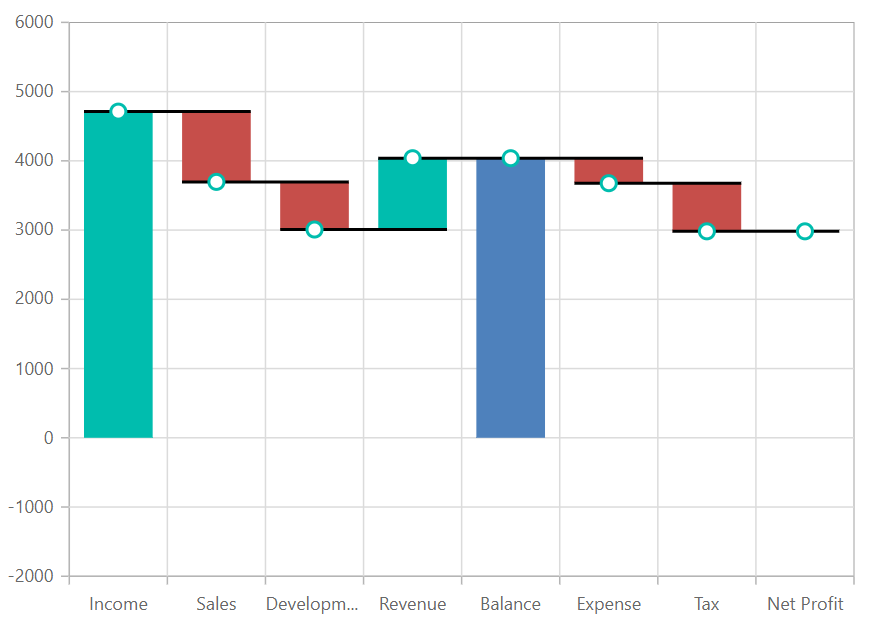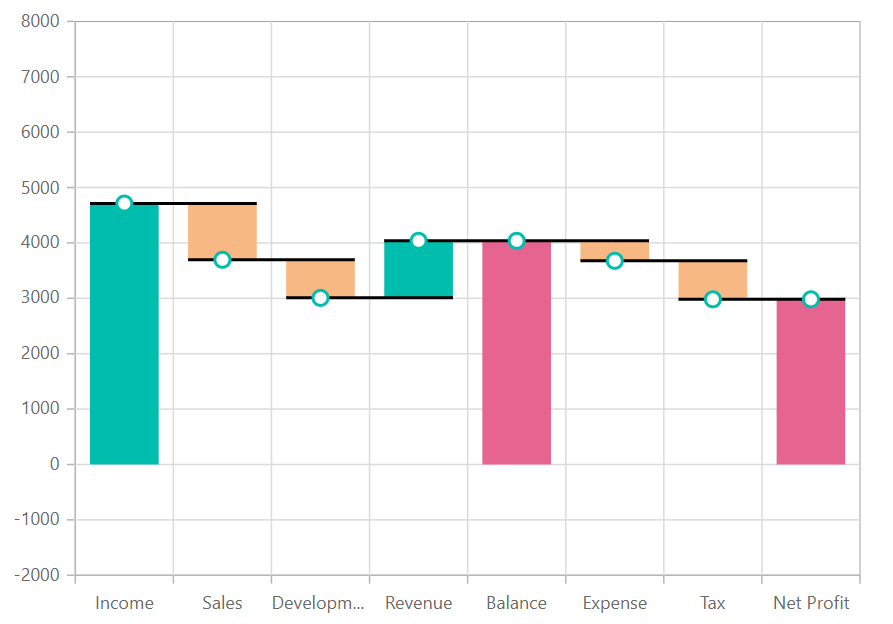# Waterfall in Blazor Charts Component

11 Aug 20233 minutes to read

## Waterfall

`Waterfall Chart` helps to understand the cumulative effect of the sequentially introduced positive and negative values. To render a waterfall series, set the series Type as Waterfall. IntermediateSumIndexes property of waterfall is used to represent the in-between sum values and SumIndexes is used to represent the cumulative sum values.

``````@using Syncfusion.Blazor.Charts

<SfChart>
<ChartPrimaryXAxis ValueType="Syncfusion.Blazor.Charts.ValueType.Category">
</ChartPrimaryXAxis>

<ChartSeriesCollection>
<ChartSeries DataSource="@SalesReports" XName="XValue" YName="YValue" Type="ChartSeriesType.Waterfall" IntermediateSumIndexes="@index" SumIndexes="@sumIndex">
<ChartMarker Height="10" Width="10" Visible="true"></ChartMarker>
</ChartSeries>
</ChartSeriesCollection>
</SfChart>

@code{
double[] index = new double[] { 4 };
double[] sumIndex = new double[] { 8 };

public class ChartData
{
public string XValue { get; set; }
public double YValue { get; set; }
}

public List<ChartData> SalesReports = new List<ChartData>
{
new ChartData { XValue = "Income", YValue = 4711 },
new ChartData { XValue = "Sales", YValue = -1015 },
new ChartData { XValue = "Development", YValue = -688 },
new ChartData { XValue = "Revenue", YValue = 1030 },
new ChartData { XValue = "Balance" },
new ChartData { XValue = "Expense", YValue = -361 },
new ChartData { XValue = "Tax", YValue = -695 },
new ChartData { XValue = "Net Profit" },
};
}``````NOTE

Explore our Blazor Waterfall Chart Example to know how to render and configure the Waterfall type chart.

## Series customization

The negative changes of waterfall charts are represented by using NegativeFillColor and the summary changes are represented by using SummaryFillColor properties respectively. By default, the NegativeFillColor is #E94649 and the SummaryFillColor is #4E81BC.

``````@using Syncfusion.Blazor.Charts

<SfChart>
<ChartPrimaryXAxis ValueType="Syncfusion.Blazor.Charts.ValueType.Category">
</ChartPrimaryXAxis>

<ChartSeriesCollection>
<ChartSeries DataSource="@ExpenseDetails" XName="XValue" SummaryFillColor="#e56590" NegativeFillColor="#f8b883"
YName="YValue" Type="ChartSeriesType.Waterfall" IntermediateSumIndexes="@intermediateSumIndexes" SumIndexes="@sumIndexes">
<ChartMarker Height="10" Width="10" Visible="true"></ChartMarker>
</ChartSeries>
</ChartSeriesCollection>
</SfChart>

@code{
double[] intermediateSumIndexes = new double[] { 4 };
double[] sumIndexes = new double[] { 7 };

public class ChartData
{
public string XValue;
public double YValue;
}
public List<ChartData> ExpenseDetails = new List<ChartData>
{
new ChartData { XValue = "Income", YValue = 4711 },
new ChartData { XValue = "Sales", YValue = -1015 },
new ChartData { XValue = "Development", YValue = -688 },
new ChartData { XValue = "Revenue", YValue = 1030 },
new ChartData { XValue = "Balance" },
new ChartData { XValue = "Expense", YValue = -361 },
new ChartData { XValue = "Tax", YValue = -695 },
new ChartData { XValue = "Net Profit" },
};
}``````NOTE

Refer to our Blazor Charts feature tour page for its groundbreaking feature representations and also explore our Blazor Chart Example to know various chart types and how to represent time-dependent data, showing trends at equal intervals.